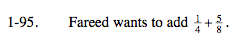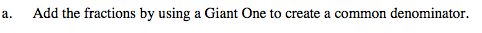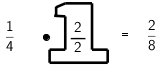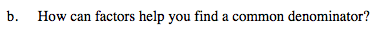### Home > MC2 > Chapter 1 > Lesson 1.2.4 > Problem1-95

1-95.$\text{What do you need to multiply } \frac{1}{4} \text{ by so that the denominator is equal to 8?}$

Multiplying the denominator by 2 gives us 8. Now use a Giant One to solve the problem.Now that both fractions have the same denominator, you can add the fractions together by adding the numerator and keeping the denominator.

$\frac{2}{8} + \frac{5}{8} = ?$Four is a factor of eight. So eight can be a common denominator.